Courses

# Nuclear Power Plant - MCQ Test 2

## 30 Questions MCQ Test GATE Mechanical (ME) 2022 Mock Test Series | Nuclear Power Plant - MCQ Test 2

Description
This mock test of Nuclear Power Plant - MCQ Test 2 for Mechanical Engineering helps you for every Mechanical Engineering entrance exam. This contains 30 Multiple Choice Questions for Mechanical Engineering Nuclear Power Plant - MCQ Test 2 (mcq) to study with solutions a complete question bank. The solved questions answers in this Nuclear Power Plant - MCQ Test 2 quiz give you a good mix of easy questions and tough questions. Mechanical Engineering students definitely take this Nuclear Power Plant - MCQ Test 2 exercise for a better result in the exam. You can find other Nuclear Power Plant - MCQ Test 2 extra questions, long questions & short questions for Mechanical Engineering on EduRev as well by searching above.
QUESTION: 1

Solution:
QUESTION: 2

### The energy released during the fission of one atom of Uranium-235 inmillion electron volts is about

Solution:

Energy released during fission of U-235 is 200 million electron volt

QUESTION: 3

### What is the function of heavy water in a nuclear reactor?

Solution:
QUESTION: 4

The most commonly used moderator in nuclear power plants is

Solution:
QUESTION: 5

With natural uranium, which of the following is used as moderator?

Solution:

Type of moderator used: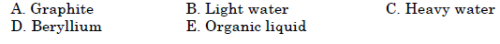QUESTION: 6

Match List-I with List-II and select the correct answer using the codes given below the Lists: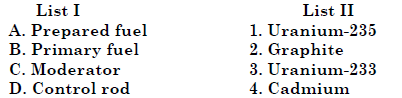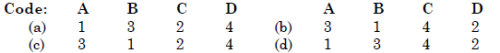Solution:
QUESTION: 7

Match List-I (Material) with List-II (Use) and select the correct answer using the codes given below the lists: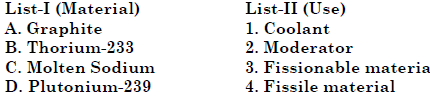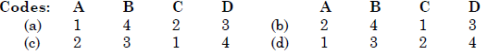Solution:
QUESTION: 8

Match List I with List II and select the correct answer.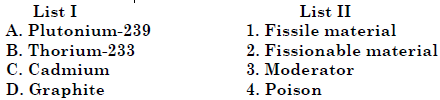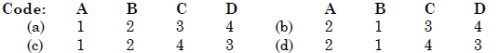Solution:
QUESTION: 9

A nuclear unit becoming critical means:

Solution:
QUESTION: 10

Consider the following statements regarding nuclear reactors:

1. In a gas-cooled thermal reactor, if CO2 is used as the coolant, a separatemoderator is not necessary as the gas contains carbon.

2. Fast reactors using enriched uranium fuel do not require a moderator.

3. In liquid metal-cooled fast breeder reactors, molten sodium is used asthe coolant because of its high thermal conductivity.

4. Fast reactors rely primarily on slow neutrons for fission.Of these statements

Solution:
QUESTION: 11

Which one of the following statements is correct?

In CANDU type nuclear reactor

Solution:
QUESTION: 12

CANDU-type nuclear reactor using natural uranium finds extensive use because
1. Heavy water is used both as coolant and moderator.
2. Cost of fuel used is much lower than that used in pressurized water or
boiling water reactor.
3. Small leakage of heavy water does not affect the performance of the
reactor substantially.
4. Fuel consumption is low because of use of heavy water.
Of these statements

Solution:
QUESTION: 13

Consider the following statements:

1. Gas cooled thermal reactors use CO2 or helium as coolant and require noseparate moderator.

2. Fast reactors use heavy water as moderator and coolant.

3. Liquid metal fast breeder reactors use molten sodium as coolant.

4. In CANDU type reactors heavy water is used as moderator.Of these correct statements are

Solution:

1. Gas cooled thermal reactors use CO2 or helium as coolant and require graphite as separate moderator.
2. Fast reactors use no moderator

QUESTION: 14

Which of the following statements are true about CANDU reactors?

1. Fuel elements contain natural-uranium dioxide

2. Pressurized heavy-water coolant is used

3. Horizontal pressure tube is used

Solution:
QUESTION: 15

The average number of fast neutrons produced in the fission of an U-235atom is nearly

Solution:
QUESTION: 16

Consider the following ways of disposal of nuclear wastes:

1. Throwing them in the deep sea.

2. Leaving them in remote, isolated open spaces in barren mountainousregions.

3. Sealing- them in concrete and depositing them in crevices and cavernsin the deep sea.

4. Storing them in sealed concrete containers in disused deep mine shafts.Safe ways of disposal of nuclear wastes would include.

Solution:
QUESTION: 17

Assertion (A): The liquid waste from a nuclear power plant is concentrated to asmall volume and stored in underground tanks.

Reason (R): Dilution of radioactive liquid waste is not desirable due to the natureof the isotopes.

Solution:
QUESTION: 18

A sodium graphite reactor uses sodium

Solution:
QUESTION: 19

What is the purpose of a moderator in a nuclear reactor?

Solution:
QUESTION: 20

The function of the moderator in a nuclear reactor is to

Solution:
QUESTION: 21

Consider the following statements:

1. Breeder reactor produces more plutonium than what it consumes.

2. Zirconium is used as a shield material

3. Lead is the commonly used moderator Of these, the ones which are not correct will include:

Solution:
QUESTION: 22

Purpose of moderator in nuclear reactor is to

Solution:
QUESTION: 23

Match List I with List II regarding nuclear reactor and select the correct answer: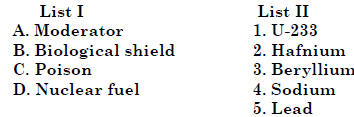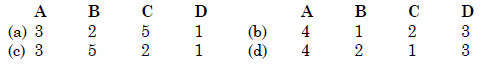Solution:
QUESTION: 24

Match List I (Component) with List II (Properties) in the context of nuclear reactors and select the correct answer using the codes given below the lists: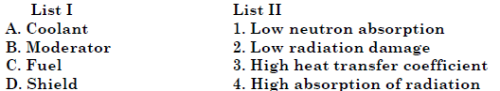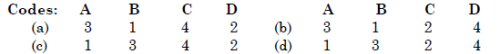Solution:
QUESTION: 25

A nuclear reactor is said to be "critical" when the neutron population inthe reactor core is

Solution:
QUESTION: 26

If k is the ratio of the rate of production of neutrons to the rate of loss of neutrons, the reactor is called a critical reactor, when

Solution:
QUESTION: 27

Which one of the following types of nuclear reactor DOES NOT require aheat exchanger?

Solution:

BWR is similar to the thermal power plant. So condenser is used no heat exchanger.

QUESTION: 28

The boiler used in the Nuclear Power Station at Tarapore, is of the

Solution:
QUESTION: 29

The moderator used in a fast breeder nuclear reactor is

Solution:
QUESTION: 30

Match List I with List II and select the correct answer using the codes given below the Lists: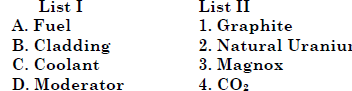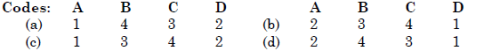Solution: